## 如何基于Cocos2d-x 3.0实现A星寻路算法 转

Software MyZone：66202765（群号，欢迎加入,若满，请加1群）
Software MyZone 1群(2dx)：286504621
【加群请写：Software MyZone或者是firedragonpzy】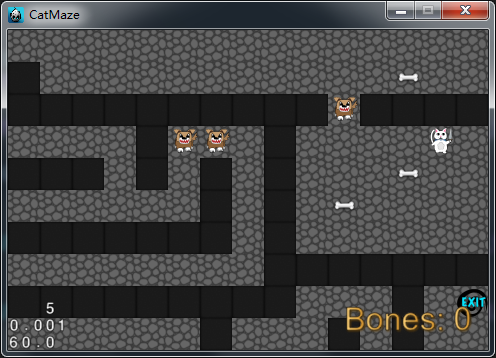## Maze猫## Maze猫和A星概览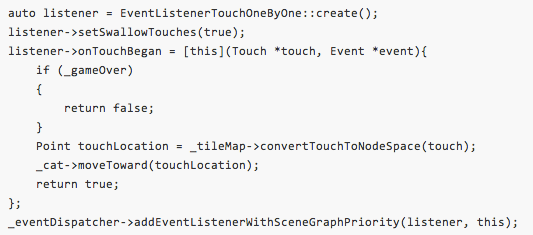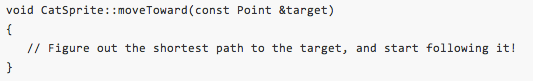`创建ShortestPathStep类`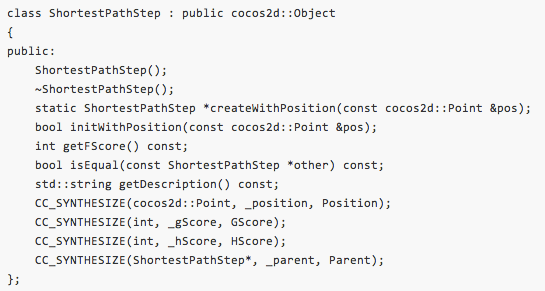```CatSprite::ShortestPathStep::ShortestPathStep() :
_position(Point::ZERO),
_gScore(0),
_hScore(0),
_parent(nullptr)
{
}
CatSprite::ShortestPathStep::~ShortestPathStep()
{
}
CatSprite::ShortestPathStep *CatSprite::ShortestPathStep::createWithPosition(const Point &pos)
{
ShortestPathStep *pRet = new ShortestPathStep();
if (pRet && pRet->initWithPosition(pos))
{
pRet->autorelease();
return pRet;
}
else
{
CC_SAFE_DELETE(pRet);
return nullptr;
}
}
bool CatSprite::ShortestPathStep::initWithPosition(const Point &pos)
{
bool bRet = false;
do
{
this->setPosition(pos);
bRet = true;
} while (0);
return bRet;
}
int CatSprite::ShortestPathStep::getFScore() const{
return this->getGScore() + this->getHScore();
}
bool CatSprite::ShortestPathStep::isEqual(const CatSprite::ShortestPathStep *other) const{
return this->getPosition() == other->getPosition();
}
std::string CatSprite::ShortestPathStep::getDescription() const{    return StringUtils::format("pos=[%.0f;%.0f]  g=%d  h=%d  f=%d",              this->getPosition().x, this->getPosition().y,                        this->getGScore(), this->getHScore(), this->getFScore());
}```

·方块的坐标

·G值（记住，这是开始点到当前点的方块数量）

·H值（记住，这是当前点到目标点的方块估算数量）

·Parent是它的上一步操作

·F值，这是方块的和值（它是G+H的值）

## 创建Open和Closed列表

```cocos2d::Vector<ShortestPathStep*> _spOpenSteps;
cocos2d::Vector<ShortestPathStep*> _spClosedSteps;```

## 检查开始和结束点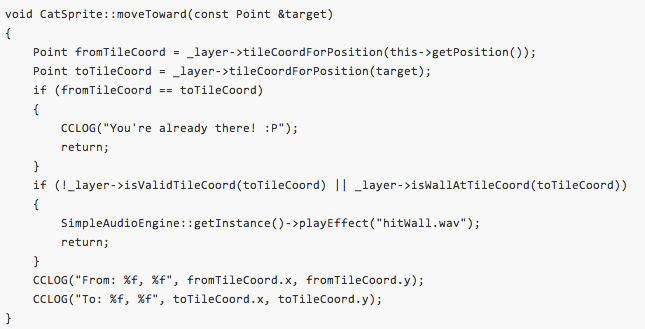```From: 24.000000, 0.000000
To: 20.000000, 0.000000```

## 实现A星算法

·一个方法用来插入一个ShortestPathStep对象到适当的位置（有序的F值）

·一个方法用来计算从一个方块到相邻方块的移动数值

·一个方法是根据”曼哈顿距离”算法，计算方块的H值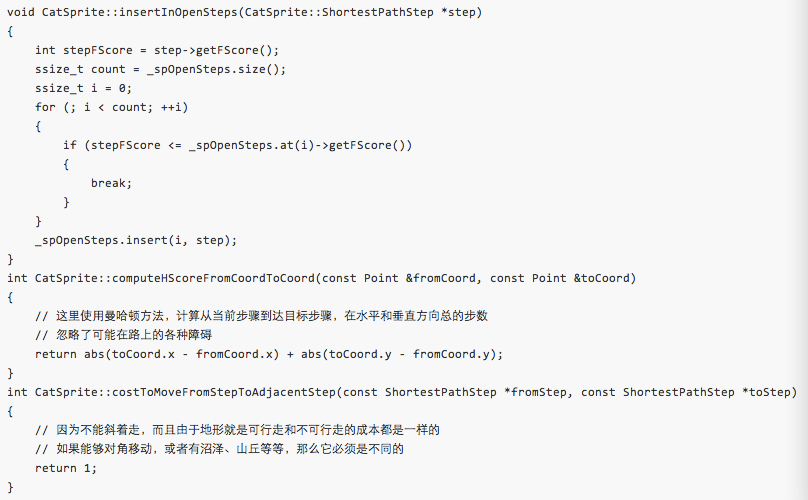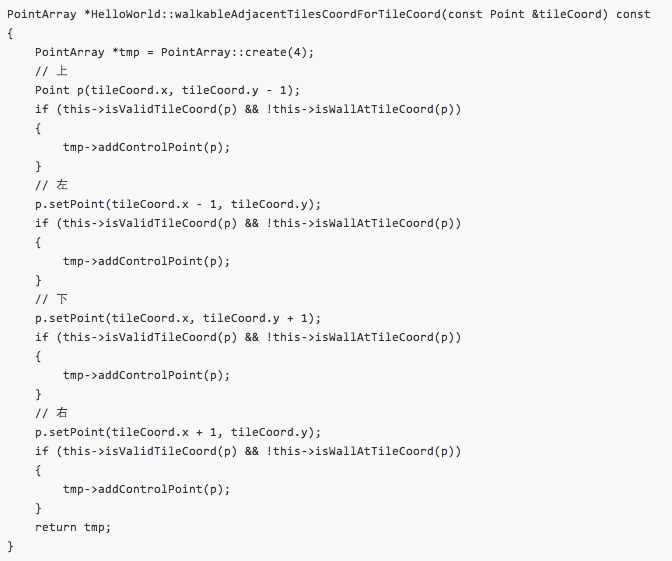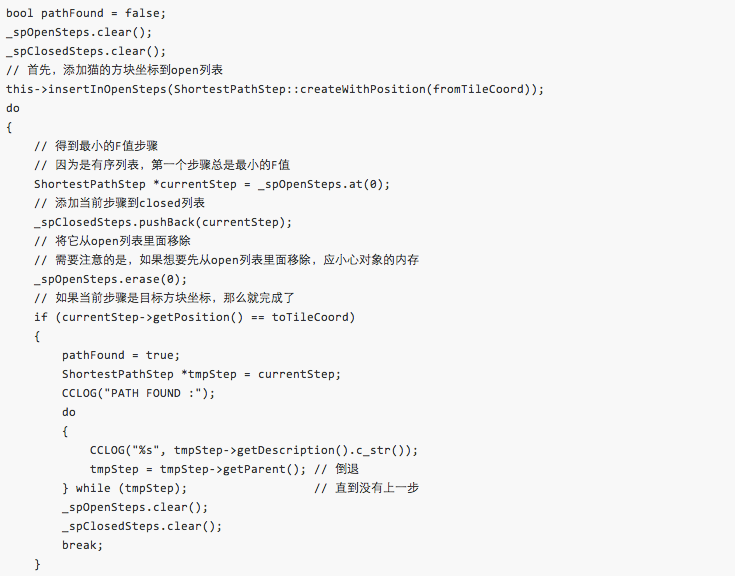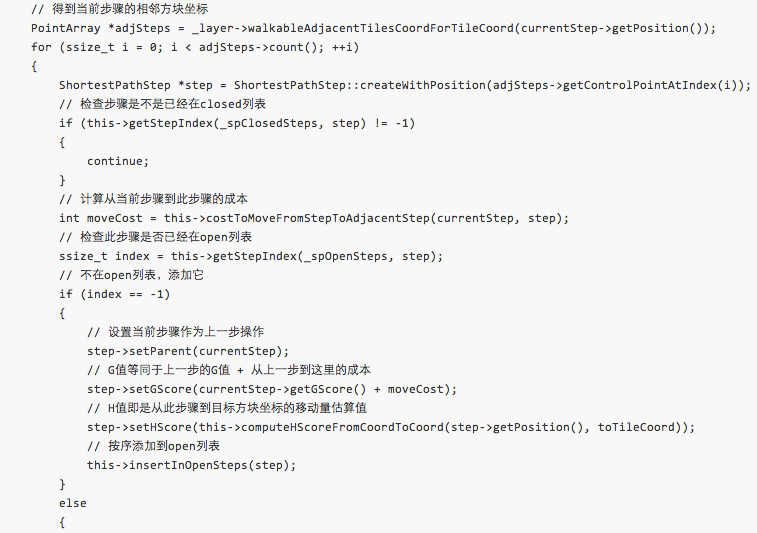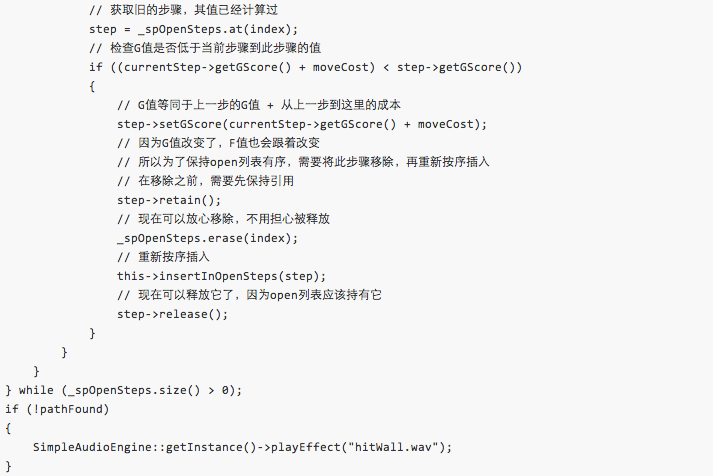```ssize_t CatSprite::getStepIndex(const cocos2d::Vector<CatSprite::ShortestPathStep *> &steps, const CatSprite::ShortestPathStep *step)
{
for (ssize_t i = 0; i < steps.size(); ++i)
{
if (steps.at(i)->isEqual(step))
{
return i;
}
}
return -1;
}``````From: 24.000000, 0.000000
To: 23.000000, 3.000000
PATH FOUND :
pos=[23;3]  g=10  h=0  f=10
pos=[22;3]  g=9  h=1  f=10
pos=[21;3]  g=8  h=2  f=10
pos=[20;3]  g=7  h=3  f=10
pos=[20;2]  g=6  h=4  f=10
pos=[20;1]  g=5  h=5  f=10
pos=[21;1]  g=4  h=4  f=8
pos=[22;1]  g=3  h=3  f=6
pos=[23;1]  g=2  h=2  f=4
pos=[24;1]  g=1  h=3  f=4
pos=[24;0]  g=0  h=0  f=0```

## 跟随路径前进

`cocos2d::Vector<ShortestPathStep*> _shortestPath;`

`//bool pathFound = false;`

```//pathFound = true;
this->constructPathAndStartAnimationFromStep(currentStep);```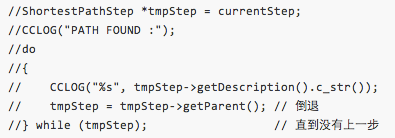```//if (!pathFound)
if (_shortestPath.empty())```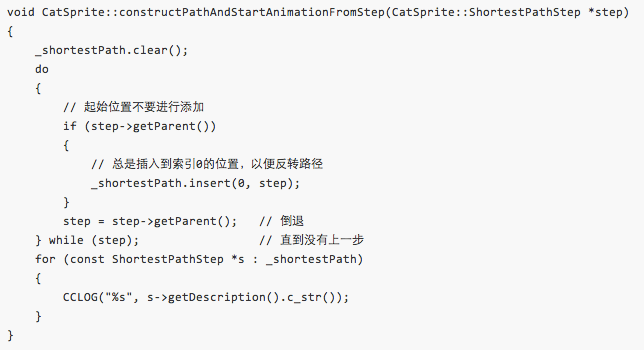```From: 24.000000, 0.000000
To: 24.000000, 3.000000
pos=[24;1]  g=1  h=2  f=3
pos=[23;1]  g=2  h=3  f=5
pos=[22;1]  g=3  h=4  f=7
pos=[21;1]  g=4  h=5  f=9
pos=[20;1]  g=5  h=6  f=11
pos=[20;2]  g=6  h=5  f=11
pos=[20;3]  g=7  h=4  f=11
pos=[21;3]  g=8  h=3  f=11
pos=[22;3]  g=9  h=2  f=11
pos=[23;3]  g=10  h=1  f=11
pos=[24;3]  g=11  h=0  f=11```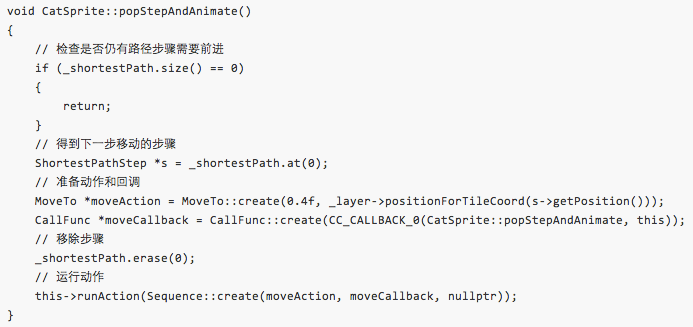`this->popStepAndAnimate();`·猫看起来有点僵硬

·猫没有带走骨头

·猫可以穿过狗（没有带着骨头），而不被吃掉

·当在猫走完路径之前，点击了一个新的路径的话，猫会有奇怪的行为

## 重新添加游戏逻辑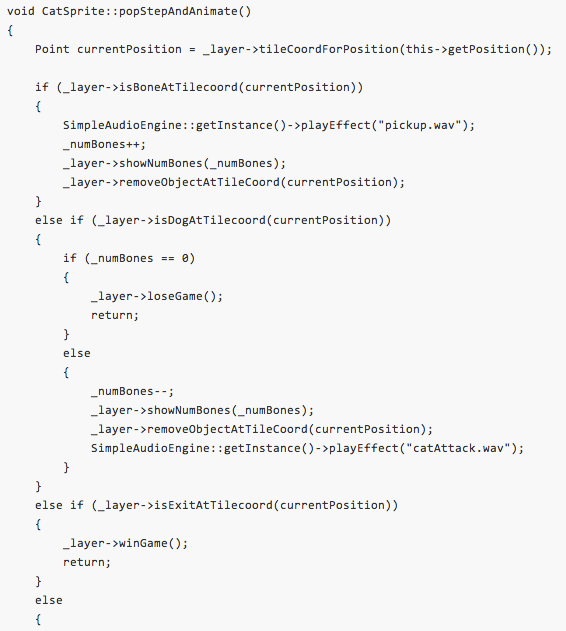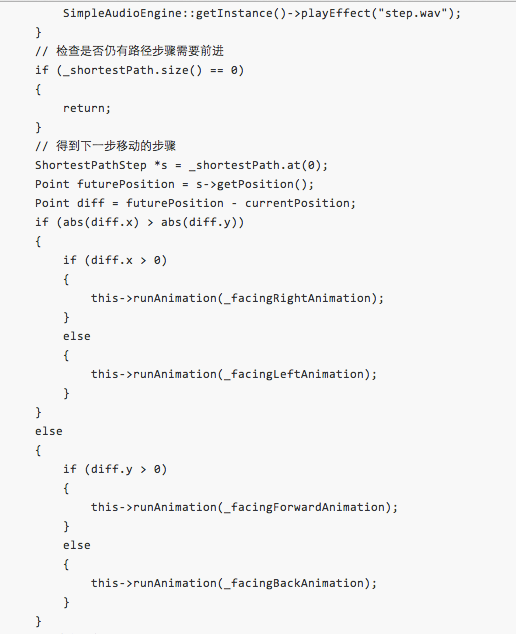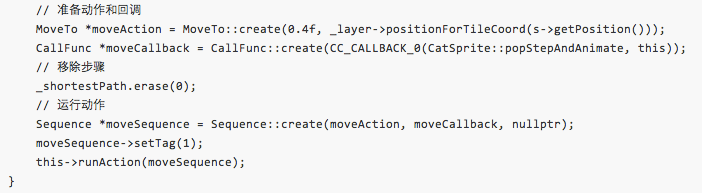`this->stopActionByTag(1);`## 如何实现对角线移动```int CatSprite::costToMoveFromStepToAdjacentStep(const ShortestPathStep *fromStep, const ShortestPathStep *toStep)
{
return ((fromStep->getPosition().x != toStep->getPosition().x)
&& (fromStep->getPosition().y != toStep->getPosition().y)) ? 14 : 10;
}```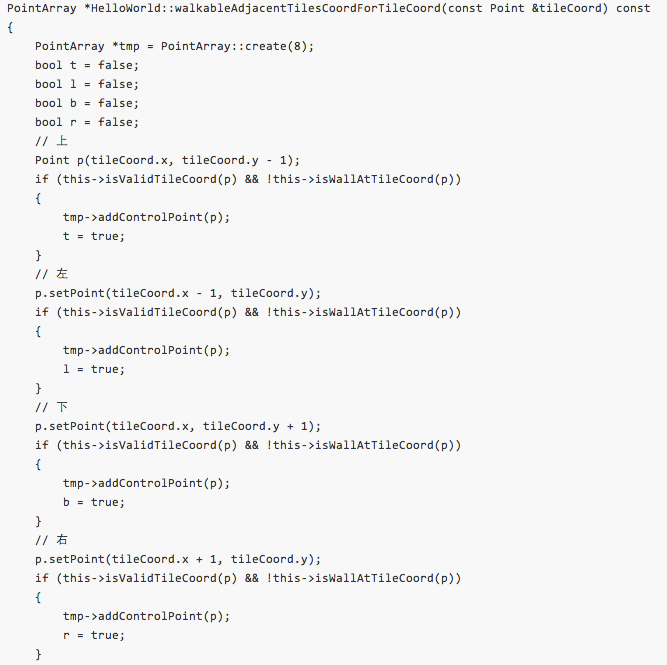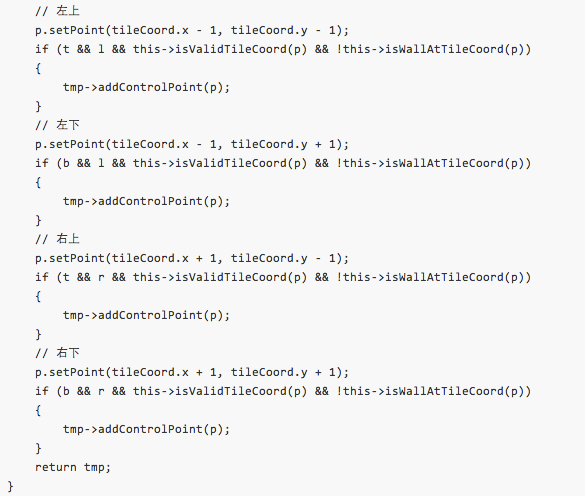·O = Origin

·T = Top

·B = Bottom

·L = Left

·R = Right

·TL = Top – Left

·…